## 5.20Transient solution controls

The following additional controls are commonly used for the transient solution described in Sec. 5.19 :

• an iterative loop around the pressure equation to update any non-orthogonal correction;
• an on/oﬀ switch for the momentum predictor.

### Non-orthogonal corrector loop

The algorithm solves the pressure equation, Eq. (5.19 ), which includes the discretisation of the Laplacian term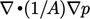, according to Sec. 3.7 . For a computational mesh with signiﬁcant non-orthogonality, the corrected scheme Eq. (3.7 ) should be applied to maintain suﬃcient accuracy.

This correction to the pressure Laplacian is calculated using the current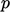, which produces an explicit contribution to the matrix equation. The equation can be imagined with the explicit correction as an additional term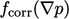.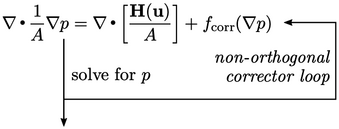To improve accuracy within each time step during a transient simulation,can be recalculated using the latest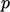and the equation solved again.

The accuracy can be improved by further iterations, but this is not generally recommended due to the increased computational cost. With the pressure equation encountered twice as part of the PISO loop, and with one corrector on each occasion, the pressure matrix is solved a total of 4 times.

Solving the pressure matrix is costly, so any reduction in the number of solutions is welcome. Recognising that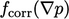is updated as part of the PISO iterations anyway, an alternative solution strategy uses no non-orthogonal correction but adds an extra PISO loop, solving the pressure equation a total of 3 times.

### Solving the momentum predictor

The transient algorithm on page 385 includes the momentum predictor that provides an initial solution for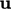, which is used to evaluate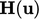in the pressure equation. The solution foris then substituted into the momentum corrector which calculates a new.

Sinceis ultimately recalculated by the momentum corrector — a momentum equation in explicit form — is it necessary to solve the momentum predictor at all? The answer is “no”, unless it makes the solution more convergent which is more likely for high speed ﬂows which are dominated more by momentum exchanges than mass conservation.

The transient algorithm should therefore include a simple switch that makes it possible to turn oﬀ the momentum predictor step. The rest of the algorithm remains the same, with the momentum matrix constructed to provideand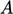.

With this switch included, the momentum predictor is then turned oﬀ in a lot of applications, e.g. highly viscous ﬂows. In practice, it is often switched oﬀ for more complex ﬂows, e.g. multiphase ﬂows, which are beyond the scope of this book.

Notes on CFD: General Principles - 5.20 Transient solution controls